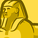Announcements
You can succeed best and quickest by helping others to succeed. Join the conversation.
cancel
Showing results for
Did you mean:Creator III

## Count of a Field condition in Set Analysis

I am using the below as my Dimension and Measure respectively.

Dimension:

if(aggr(RANK(Count(distinct{\$<[Group]={'A'}>} ID)),[Product ])<=10,[Product ])

Measure:

=Num(Count(Distinct {<[Group]={'A'}, [Date]={"\$(='>=' & Date(AddMonths(Max([Date]),-13)) & '<=' & Date(Max([Date]))))"}>} ID)
/
Count(Distinct {<[Group]=>} ID)
,'#,##0%')

This is to show Top 10 products and their respective % as a Measure. Now I have additional requirement where I need to show only those products in the chart that have Count(Distinct ID) >=50.  I am not sure where and how to include this in Dimension/Measure. I tried using Product ={"=Count(Distinct ID)>=50 "} in Dimension/Measure but that's not helping.

Labels (4)

• ### VisualizationMVP

Try this

if(aggr(RANK(Count({< [Group]={'A'} >} ID)), [Product]) <= 10

and Count({< [Group]={'A'} >} ID) >= 50, [Product]

)Tags
Community Browser